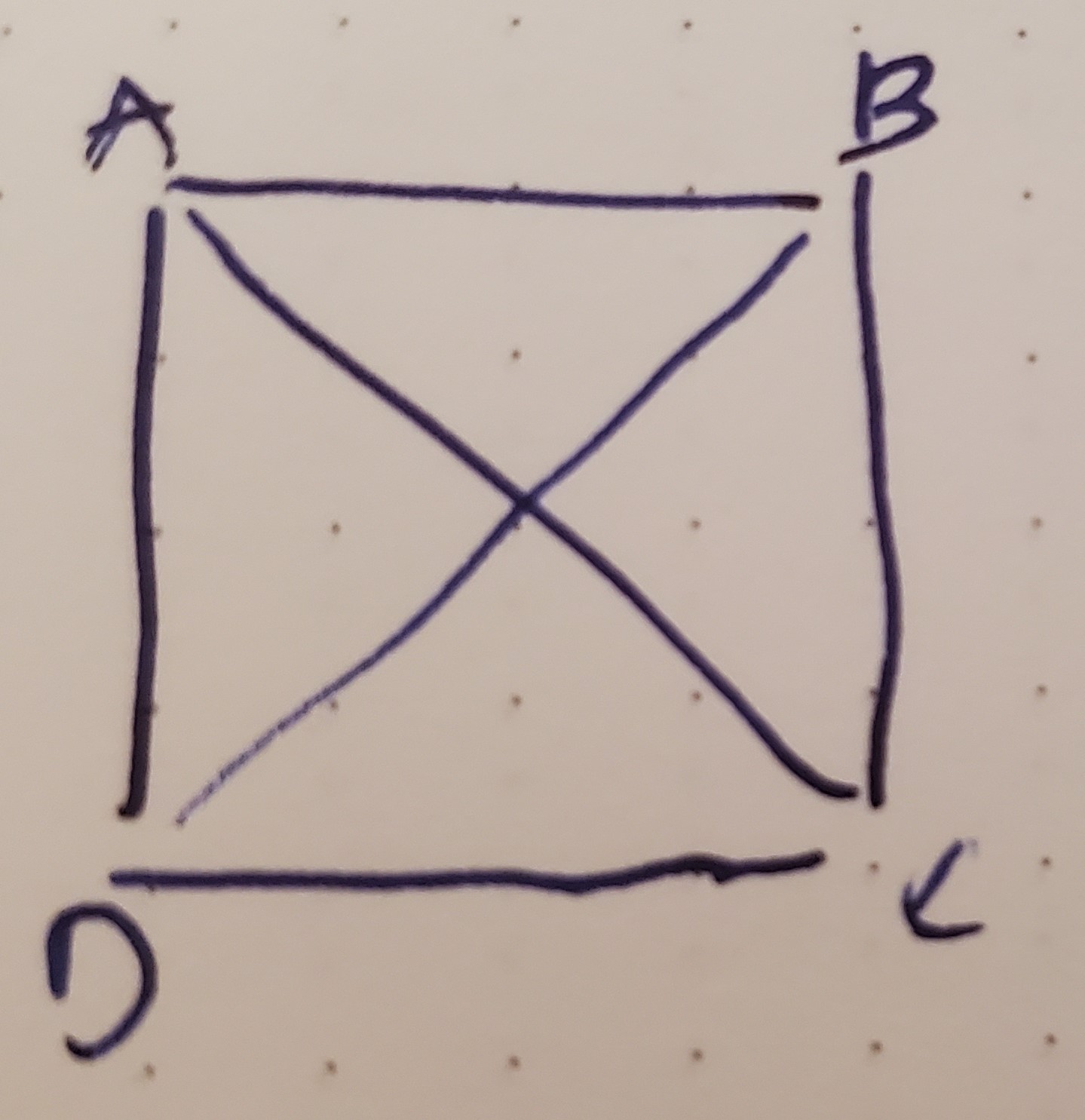I told you I’d do it, so I did it. I re-solved Challenge #123 Task 2.

To reiterate, we have four points: A, B, C and D.There are six possible lines: A→B, A→C, A→D, B→C, B→D and C→D. (I assert that A→C is equivalent to C→A.) For a square, the lines A→C and B→D would be

• the longest two lines and
• the same length

So, the steps for a `is_square` test?

• Check that there are four points. A triangle cannot be a square. A pentagon cannot be a square.
• (For us), make the points Points.
• make lines between every two points.
• sort the lines by length (with the method `distance` discussed previously).
• If the four shortest are equally long, then you have a square or a parallelogram. If the two longest are equally long, then you have a square or a rectangle. So you need to check both.

So, that code. I’ll point out that I used the randomizing sort — `sort { rand 10 <=> rand 10 }` — to ensure that the written order of the data is not the order of points as considered.

``````#!/usr/bin/env perl

use strict;
use warnings;
use feature qw{ say signatures state };
no warnings qw{ experimental };

use Cwd qw( abs_path );
use File::Basename qw( dirname );
use lib dirname( abs_path(\$0) );
use CorLine;
use CorPoint;

use JSON;

my \$json = JSON->new->canonical;

my @data = (
[ [ 10, 20 ], [ 20, 20 ], [ 20, 10 ],  [ 10,  10 ], ],
[ [ 12, 24 ], [ 16, 10 ], [ 20, 12 ],  [ 18,  16 ], ],
[ [ 40, 40 ], [ 50, 30 ], [ 40, 20 ],  [ 30,  30 ], ],
[ [ 10, 10 ], [ 15, 15 ], [ 20, 15 ],  [ 15,  5 ], ],
[ [ 00, 10 ], [ 10, 00 ], [ 00, -10 ], [ -10, 0 ], ],
[ [ 01, 03 ], [ -3, 01 ], [ -1, -3 ],  [ 03,  -1 ] ],
);

for my \$d (@data) {
\$d->@* = sort { rand 10 <=> rand 10 } \$d->@*;
say \$json->encode(\$d);
say is_square( \$d->@* ) ? 'square' : 'not square';
say '';
}

sub is_square ( @base ) {
return 0 unless scalar @base == 4;
my @points;
my @lines;
for my \$xy (@base) {
my ( \$x, \$y ) = \$xy->@*;
push @points, CorPoint->new( x => \$x, y => \$y );
}

for my \$i ( 0 .. 2 ) {
for my \$j ( \$i + 1 .. 3 ) {
push @lines, CorLine->new( i => \$points[\$i], j => \$points[\$j] );
}
}

my @shortest = sort { \$a->dist <=> \$b->dist } @lines;
\$#shortest = 3;

my @longest = sort { \$b->dist <=> \$a->dist } @lines;
\$#longest = 1;

return 1
if \$shortest->dist == \$shortest->dist
and \$shortest->dist == \$shortest->dist
and \$shortest->dist == \$shortest->dist
and \$shortest->dist == \$shortest->dist
and \$longest->dist == \$longest->dist;
return 0;
}
``````
``````[[20,20],[20,10],[10,10],[10,20]]
square

[[18,16],[12,24],[16,10],[20,12]]
not square

[[5,3],[3,3],[4,2],[4,4]]
square

[[15,15],[10,10],[20,15],[15,5]]
not square

[[0,10],[10,0],[-10,0],[0,-10]]
square

[[-1,-3],[-3,1],[3,-1],[1,3]]
square
``````